Next: Conclusion Up: number10 Previous: Fitting data to a

A nonlinear example

In the above example, we could solve for the optimal set of parameters because we could separate out the equations to give one just for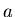and one just for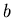. In order to do this, the cost function must be linear with respect to the parameters, that is the parameters do not show up as nonlinear functions of each other ( e.g.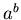, or within transcendental functions, such a cosine). Sometimes this linearity can be achieved by applying a transformation to the original problem to arrive at a new problem which is linear. For example, consider the problem of estimating the amplitude and phase of a signal at a known frequency,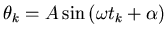(9)

where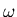is known,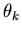and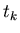are observed and where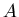and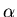are to be estimated.

The simplest cost function would be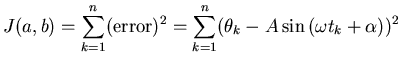(10)

Our equations now give us,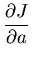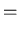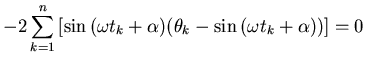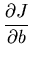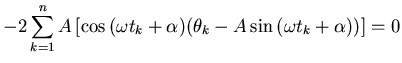These equations are hopelessly intertwined, theterms cannot be pulled out into an equation forthat is separate from thein the same way that we achieved (7) and (8). The direct solution of these equations requires an approximation involving successive iterations or some other method.

This problem fortunately has the nice property that it can be transformed into another estimation problem which is linear. If we let,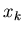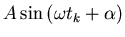(11)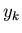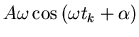(12)

then with a little trigonometry, we can write our unknownsandas,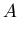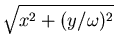(13)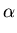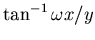(14)

(as long as the amplitude and phase are constant, it does not matter where in our data set we apply (13) and (14), so we drop the indexing subscripts). Now can we find an optimal estimate of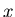and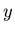? If so, then we are all set. It turns out that we can. The complicating factor here is that our cost function must now account for the two componentsand, while we still are relying on the observations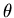.

With lots of messy, tedious, but straightforward algebra we can solve this problem to arrive at: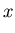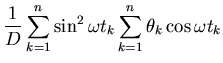(15)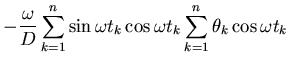(16)

for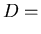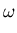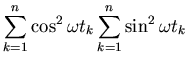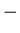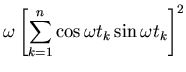How does one come up with this kind of transformation for a new problem ? There are a few fairly standard transformations that you can use, for instance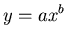can be converted to a straight line fit problem by taking the logarithm of both sides. But mostly, finding a suitable transformation is a matter of experiance, persistance and luck.Next: Conclusion Up: number10 Previous: Fitting data to a
Skip Carter 2008-08-20